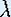Up: Bifurcation

# One-Dimensional Dynamical Systems

## Part 5: Bifurcation

#### Bifurcation points

The Logistic map has an attracting fixed point for= 1.5 that becomes repelling as we increaseto 3.1.

Definition (Bifurcation Point): In case of a bifurcation, there is a special valueb for which the following holds: the dynamics forclose to but smaller thanb is qualitatively different from the dynamics forclose to but larger thanb This valueb is called a bifurcation point.

Not every value ofis a bifurcation point. Only when a qualitative change in the dynamics occurs, we say that the function underwent a bifurcation.

• Approximately at whatvalue between 1.5 and 3.1 does a bifurcation occur?

• At exactly whatvalue between 1.5 and 3.1 does a bifurcation occur? Explain why you know this value is exact.

In the following section we show how to visualize the dynamics as a function of.

Up: BifurcationThe Geometry Center Home Page

Written by Hinke Osinga